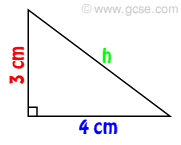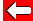# Pythagoras in Action

Here's a triangle with the lengths of its two shortest sides known: 3 and 4 cm. We can use Pythagoras's theorem to find the length of the hypotenuse:hypotenuse² = one-side² + second-side²

h² = 3² + 4²

h² = 9 + 16 = 25

h = 5 cm

Let's see if you can do it yourself.Go back a pageMaths MenuGo to next page